## TL;DR Contrary to popular belief, Venus is not Earth's closes...
CGP Grey did an [interesting video](https://www.youtube.com/watch?v...
![](https://i.imgur.com/UxUaFj9.png) This diagram illustrates the ...
Could you provide a link to elliptical integrals, generally, and on...
![](https://www.flother.is/processed_images/ee27b186415fe87e00.png)...
Here's the link to the BBC episode of [More or Less](https://www.bb...
It should say Figure 4 instead of Figure 3 in comparing the results...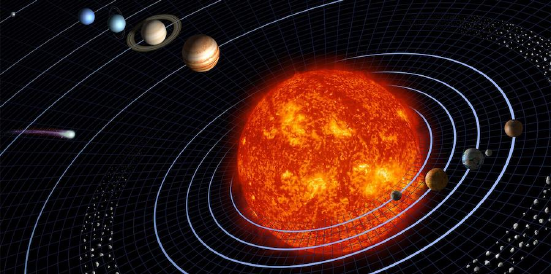Venus is not Earth’s closest neighbor
Tom Stockman, Gabriel Monroe, and Samuel Cordner
12 March 2019
Abstract
Calculations and simulations conﬁrm that on average, Mercury is the
nearest planet to Earth—and to every other planet in the solar system.
Figure 1: An illustration of the solar system. Credit: NASA
1 Introduction
Which planet is closest to Earth? Ask an astronomer or a search engine, and
you’ll probably hear that though the situation changes frequently, Venus is
the closest when averaged over time. Several educational websites, such as The
Planets and Space Dictionary, publish the distance between each pair of planets,
and they all show that Venus is nearest to Earth on average. They’re all wrong.
NASA literature even tells us Venus is “our closest planetary neighbor,” which
is true if we are talking about which planet has the closest approach to Earth
but not if we want to know which planet is closest on average.
As it turns out, by some phenomenon of carelessness, ambiguity, or group-
think, science popularizers have disseminated information based on a ﬂawed
assumption about the average distance between planets. Using a mathematical
method that we devised, we determine that when averaged over time, Earth’s
nearest neighbor is in fact Mercury.
1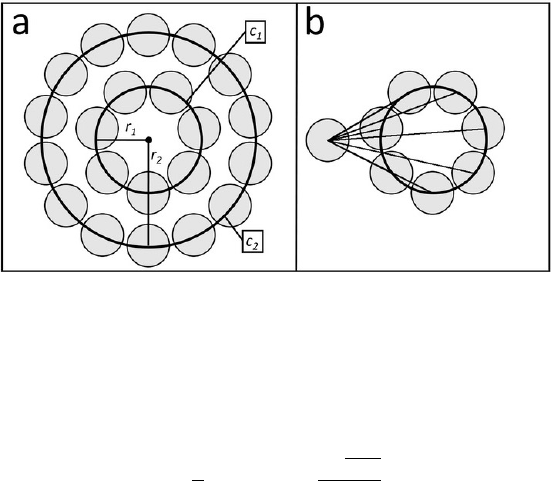2 Simple but wrong
To calculate the average distance between two planets, The Planets and other
websites assume the orbits are coplanar and subtract the average radius of the
inner orbit, r
1
, from the average radius of the outer orbit, r
2
. The distance
between Earth (1 astronomical unit from the Sun) and Venus (0.72 AU) comes
out to 0.28 AU.
Although it feels intuitive that the average distance between every point
on two concentric ellipses would be the diﬀerence in their radii, in reality that
diﬀerence determines only the average distance of the ellipses’ closest points.
3 A better approach
To more accurately capture the average distance between planets, we devised
the point-circle method (PCM). The PCM treats the orbits of two objects as
circular, concentric, and coplanar. An object in a circular orbit maintains con-
stant velocity, which means that over a suﬃciently long period, it is equally
likely to be in any position in that orbit.
Figure 2: (a) The uniform probabilistic distribution of two circularly orbiting
1
and r
2
. (b) Radial symmetry is used to simplify
the average distance between the distributions.
It can be determined by integrating the point-to-point distance around c
1
,
as simpliﬁed in the following equation, which deﬁnes the PCM:
¯
d =
2
π
(r
1
+ r
2
)E
2
r
1
r
2
r
1
+ r
2
(1)
where E(x) is an elliptic integral of the second kind. The average distances
between pairs of planets determined by the PCM are included in the table.
Venus is on average 1.14 AU from Earth, but Mercury is a much closer 1.04 AU.
From the PCM, we noticed that the distance between two orbiting bodies is
at a minimum when the inner orbit is at a minimum. That observation results in
2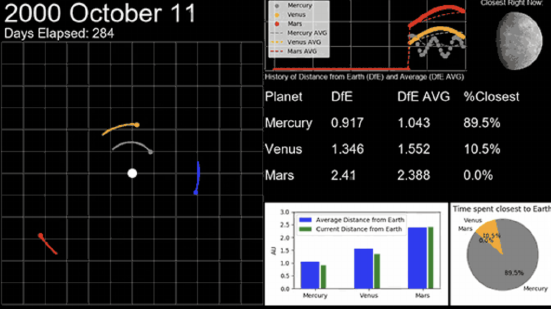Figure 3: A simulation of an Earth year’s worth of orbits by the terrestrial
planets begins to reveal that Mercury (gray in orbital animation) has the small-
est average distance from Earth (blue) and is most frequently Earth’s nearest
neighbor. A longer run of the simulation can be seen on YouTube. In addition,
planetary geoscientist David Rothery ran a solar system simulation for the BBC
radio program More or Less and came up with similar results.
what we call the whirly-dirly corollary (named after an episode of the cartoon
Rick and Morty): For two bodies with roughly coplanar, concentric, circular
orbits, the average distance between the two bodies decreases as the radius of
the inner orbit decreases. It’s clear from this corollary, and from the table,
that Mercury (average orbital radius of 0.39 AU), not Venus (average radius of
0.72 AU), is the closest planet to Earth on average. In fact, Mercury is even
the closest planet to Neptune. (And yes, to Pluto too: Though the corollary
doesn’t work as well for the dwarf planet, with its orbital inclination of 17° and
eccentricity of 0.25, its nearest neighbor is also Mercury.)
4 Simulation validation
We ran a simulation to conﬁrm the whirly-dirly corollary, using a Python library
called PyEphem to chart the positions of all eight planets in the solar system
for 10 000 years. An animation illustrating the simulation is shown in ﬁgure
2. After each 24 hours of simulated time, the program records the distances
between each pair of planets.
In the table we list the average measured distances over the 10 millennia
and compare them with the results from the PCM and the traditional method.
The simulation results diﬀer from the ﬂawed numbers by up to 300 %, but
they deviate from the PCM ﬁgures by less than 1 %. Figure 3 compares the
results from both methods with the simulation of the average distance between
Neptune and the seven other planets.
3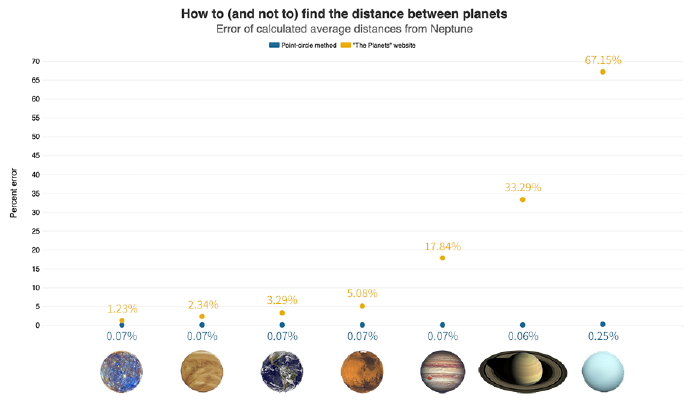Figure 4: The point-circle method (blue points) calculates the average dis-
tance from Neptune to the other seven planets far more accurately than the
method used by websites such as The Planets (yellow). The values are com-
pared with those obtained from the 10000-year solar system simulation. Credit:
Greg Stasiewicz and Flourish
5 Conclusion
As best we can tell, no one has come up with a concept like PCM to compare
orbits. With the right assumptions, PCM could possibly be used to get a quick
estimate of the average distance between any set of orbiting bodies. Perhaps it
can be useful for quickly estimating satellite communication relays, for which
signal strength falls oﬀ with the square of distance. In any case, at least we know
now that Venus is not our closest neighbor—and that Mercury is everybody’s.
4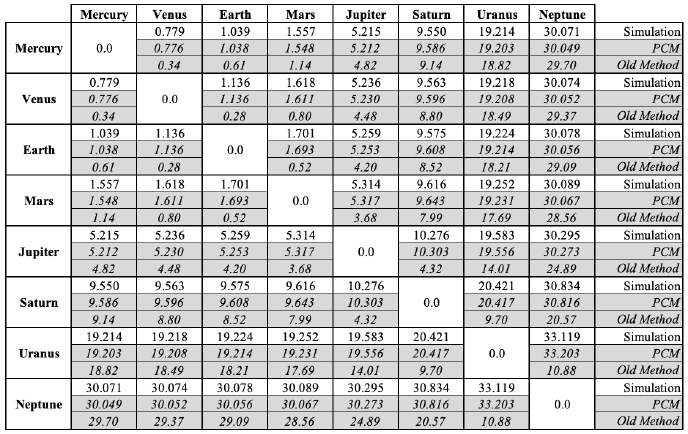Figure 5: A comparison of the average distances (in astronomical units) between
planets, as obtained via simulation, the point-circle method, and the common
method used by various educational websites. The PCM results are far closer to
those from the simulation, correctly demonstrating that Mercury is every other
planet’s closest neighbor.
5
## TL;DR Contrary to popular belief, Venus is not Earth's closest neighbor on average. This misunderstanding stems from a common but flawed assumption about the average distance between planets. Averaged over time, Mercury is actually the closest planet to Earth — and to every other planet in the solar system. This new approach can potentially improve our understanding of orbital dynamics and could be useful in estimating satellite communication relays. ![](https://www.flother.is/processed_images/ee27b186415fe87e00.png) You can actually use this [python script](https://github.com/flother/nearest-planet) by Matt Rigott to calculate which planet will be our nearest neighbour over the coming months and years! Here's the link to the BBC episode of [More or Less](https://www.bbc.co.uk/programmes/m0001y9p). CGP Grey did an [interesting video](https://www.youtube.com/watch?v=SumDHcnCRuU) on this topic. It should say Figure 4 instead of Figure 3 in comparing the results from both methods with the simulation of the average distance between Neptune and the seven other planets. Could you provide a link to elliptical integrals, generally, and one for elliptic integrals of the second kind, so that we could learn how equation (1) was obtained. Thank you. ![](https://i.imgur.com/UxUaFj9.png) This diagram illustrates the terms used in the point-circle problem. The equation for distance in a radial coordinate system is $$\text {d}=\sqrt{r_1^2+r_2^2+r_1 r_2 \cos (\theta)}$$ The equation for finding the average value of a function is $$\text { Average Value }=\frac{1}{b-a} \int_a^b f(x) d x$$ Substituting $f(x)$ with Distance $(\theta)$ from the previous equation and integrating from 0 to $2 \pi$ yields $$\text { Average Distance }=\frac{1}{2 \pi} \int_0^{2 \pi} \sqrt{\left(r_1^2+r_2^2+r_1 r_2 \cos (\theta)\right.} d \theta$$ which can be simplified using a complete elliptic integral of the second kind. $$\text { Average Distance }=\frac{2}{\pi}\left(r_1+r_2\right) E\left(\frac{2 \sqrt{r_1 r_2}}{r_1+r_2}\right)$$ where $E()$ is an elliptic integral of the second kind. The use of this last equation for calculating the average distance between 2 orbiting bodies will hence be referred to as the Point-Circle Method (PCM). The average distances between pairs of planets determined by the PCM are shown as part of Figure 6. The Whirly Dirly Corollary Plotting the last equation reveals an interesting behavior. The distance between two orbiting bodies is at a minimum when the inner orbit is at a minimum. This observation results in the Whirly Dirly Corollary (WDC): for two bodies with roughly concentric circular orbits around a common center, the average distance between the two bodies decreases as the radius of the inner orbit decreases. From this corollary it is observed that Mercury (average orbital distance of 0.39 AU), not Venus (average orbital distance of $0.72 \mathrm{AU}$ ), is the closest planet to Earth on average.